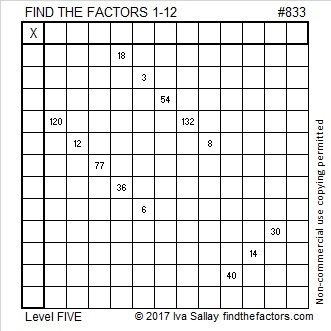# 833 and Level 5Print the puzzles or type the solution on this excel file: 12 factors 829-834

Wrap Your Mind Around This:  × 17 = 833 = 28² + 7²

833 is the hypotenuse of a Pythagorean triple:

• 392-735-833 calculated from 2(28)(7), 28² – 7², 28² + 7²

833 can be written as the sum of consecutive prime numbers two ways. One of those ways starts with one of its prime factors, 17:

• 833 is the sum of the seventeen prime numbers from 17 to 83
• 833 is also the sum of the eleven prime numbers from 53 to 101

Since 833 has three factor pairs where both factors are odd, it can be written as the difference of two squares three different ways:

• 833 × 1 = 833 means 417² – 416² = 833
• 119 × 7 = 833 means 63² – 56² = 833
• 49 × 17 = 833 means 33² – 16² = 833

The 41st triangular number will be 861. We must use less than 41 consecutive numbers if we want to express 833 as the sum of consecutive numbers. 833 has 3 odd factors (1, 7, 17) less than 41. Thus 833 can be written as the sum of 7 consecutive numbers and as the sum of 17 consecutive numbers. Notice 833’s factor pairs below highlighted in red.

• 833 = 116 + 117 + 118 + 119 + 120 + 121 + 122; that’s 7 consecutive numbers
• 833 = 41 + 42 + 43 + 44 + 45 + 46 + 47 + 48 + 49 + 50 + 51 + 52 + 53 + 54 + 55 + 56 + 57; that’s 17 consecutive numbers

The factor of 833 that is the highest power of 2 is 1 because 2º=1. Each of those odd factors, (1, 7, 17), times 2 × 1 is still less than 40, so 833 can also be written as the sum of 2 consecutive numbers, the sum of 14 consecutive numbers, and the sum of 34 consecutive numbers:

• 833 = 416 + 417; that’s 2 consecutive numbers
• 833 = 53 + 54 + 55 + 56 + 57 + 58 + 59 + 60 + 61 + 62 + 63 + 64 + 65 + 66; that’s 14 consecutive numbers
• 833 = 8 + 9 + 10 + 11 + 12 + 13 + 14 + 15 + 16 + 17 + 18 + 19 + 20 + 21 + 22 + 23 + 24 + 25 + 26 + 27 + 28 + 29 + 30 + 31 + 32 + 33 + 34 + 35 + 36 + 37 + 38 + 39 + 40 + 41; that’s 34 consecutive numbers

Here is 833’s factoring information:

• 833 is a composite number.
• Prime factorization: 833 = 7 × 7 × 17, which can be written 833 = 7² × 17
• The exponents in the prime factorization are 2 and 1. Adding one to each and multiplying we get (2 + 1)(1 + 1) = 3 × 2  = 6. Therefore 833 has exactly 6 factors.
• Factors of 833: 1, 7, 17, 49, 119, 833
• Factor pairs: 833 = 1 × 833, 7 × 119, or 17 × 49
• Taking the factor pair with the largest square number factor, we get √833 = (√49)(√17) = 7√17 ≈ 28.861739